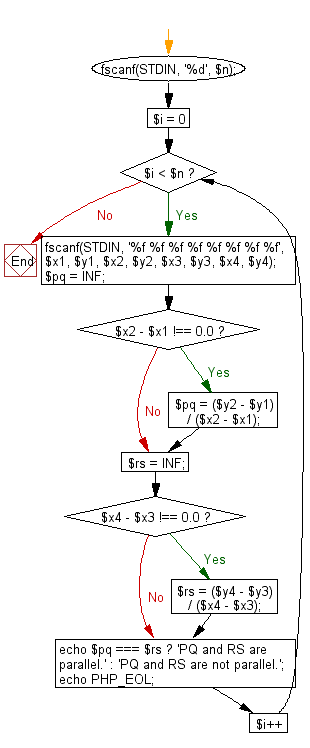﻿ PHP Exercise: Test whether two lines PQ and RS are parallel - w3resource# PHP Exercises: Test whether two lines PQ and RS are parallel

## PHP: Exercise-56 with Solution

Write a PHP program to test whether two lines PQ and RS are parallel.
The four points are P(x1, y1), Q(x2, y2), R(x3, y3), S(x4, y4).

Input: −100 ≤ x1, y1, x2, y2, x3, y3, x4, y4 ≤ 100
Each value is a real number with at most 5 digits after the decimal point.

Sample Solution: -

PHP Code:

``````<?php
fscanf(STDIN, '%d', \$n);
for (\$i = 0; \$i < \$n; \$i++) {
fscanf(STDIN, '%f %f %f %f %f %f %f %f', \$x1, \$y1, \$x2, \$y2, \$x3, \$y3, \$x4, \$y4);
\$pq = INF;
if (\$x2 - \$x1 !== 0.0) {
\$pq = (\$y2 - \$y1) / (\$x2 - \$x1);
}
\$rs = INF;
if (\$x4 - \$x3 !== 0.0) {
\$rs = (\$y4 - \$y3) / (\$x4 - \$x3);
}
echo \$pq === \$rs ? 'PQ and RS are parallel.' : 'PQ and RS are not parallel.';
echo PHP_EOL;
}
?>
```
```

Sample Input:
2
1.0 0.0 3.0 2.0 2.0 2.0 0.0 0.0
4.0 3.0 10.0 7.0 14.0 5.0 8.0 10.0

Sample Output:

```PQ and RS are parallel.
PQ and RS are not parallel.
```

Flowchart:PHP Code Editor:

Have another way to solve this solution? Contribute your code (and comments) through Disqus.

What is the difficulty level of this exercise?

﻿

New Content: Composer: Dependency manager for PHP, R Programming